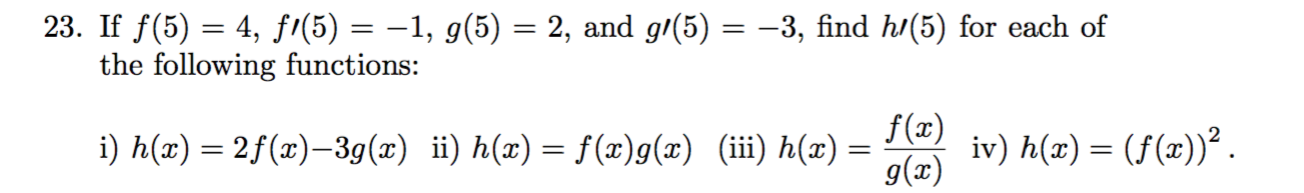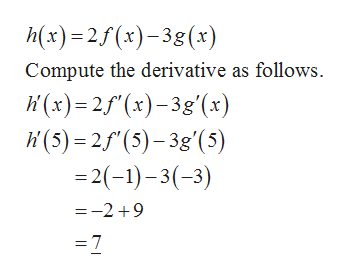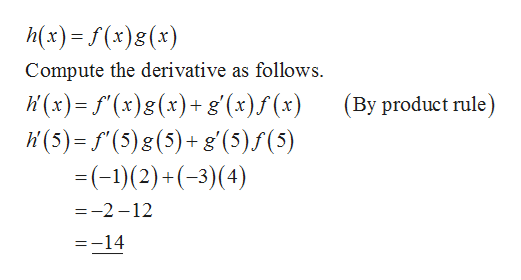# 23. If f(5) = 4, fi(5) = –1, g(5) = 2, and g/(5) = -3, find h/(5) for each ofthe following functions:f(x)iv) h(x) = (f(x))².g(x)i) h(x) = 2f(x)–3g(x) ii) h(x) = f(x)g(x) (iii) h(x) =

Question
22 viewshelp_outlineImage Transcriptionclose23. If f(5) = 4, fi(5) = –1, g(5) = 2, and g/(5) = -3, find h/(5) for each of the following functions: f(x) iv) h(x) = (f(x))². g(x) i) h(x) = 2f(x)–3g(x) ii) h(x) = f(x)g(x) (iii) h(x) = fullscreen
check_circle

Step 1

Given:

Step 2

i) The given function is,help_outlineImage Transcriptioncloseh(x)=25(x)-38(x) Compute the derivative as follows. W (x)= 2f"(x)-3g'(x) K (5) = 2f"(5)– 3g'(5) = 2(-1)– 3(-3) =-2 +9 =7 fullscreen
Step 3

ii) The given fun...help_outlineImage Transcriptioncloseh(x) = f (x)g(x) %3D Compute the derivative as follows. K(x)= f'(x)g(x)+g'(x)f (x) li (5) = f"(5) g(5)+ g'(5)f(5) =(-1)(2) +(-3)(4) (By product rule) =-2 -12 =-14 fullscreen

### Want to see the full answer?

See Solution

#### Want to see this answer and more?

Solutions are written by subject experts who are available 24/7. Questions are typically answered within 1 hour.*

See Solution
*Response times may vary by subject and question.
Tagged in

### Derivative